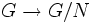# Inflation functor on cohomology

Suppose$G$ is a group and$N$ is a normal subgroup of$G$. Suppose$A$ is an abelian group and$\varphi:G \to \operatorname{Aut}(A)$ is a homomorphism of groups, making$A$ into a$G$-module. Denote by$A^N$ the subgroup of$A$ fixed pointwise by all elements of$N$.
Then, the inflation homomorphism$\operatorname{inf}: H^*(G/N;A^N) \to H^*(G;A)$ is defined as the composite:$H^*(G/N;A^N) \stackrel{\operatorname{res}}{\to} H^*(G;A^N) \to H^*(G;A)$
where the first map is the restriction homomorphism$H^*(G/N;A^N) \to H^*(G;A^N)$ corresponding to the quotient map$G \to G/N$ and the second map is the natural map obtained by viewing cohomology as a covariant functor in its second coordinate, applied to the injection$A^N \to A$ of$G$-modules.